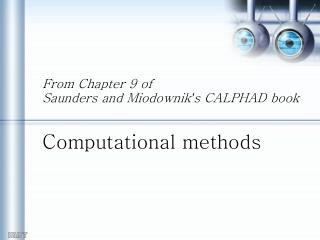Download PresentationFrom Chapter 9 of Saunders and Miodownik ’ s CALPHAD book Computational methodsFrom Chapter 9 of Saunders and Miodownik ’ s CALPHAD book Computational methods - PowerPoint PPT Presentation

Download PresentationFrom Chapter 9 of Saunders and Miodownik ’ s CALPHAD book Computational methods
An Image/Link below is provided (as is) to download presentation

Download Policy: Content on the Website is provided to you AS IS for your information and personal use and may not be sold / licensed / shared on other websites without getting consent from its author. While downloading, if for some reason you are not able to download a presentation, the publisher may have deleted the file from their server.

- - - - - - - - - - - - - - - - - - - - - - - - - - - E N D - - - - - - - - - - - - - - - - - - - - - - - - - - -
Presentation Transcript

1. From Chapter 9 of Saunders and Miodownik’s CALPHAD bookComputational methods

2. for many years: equil const used to express (the abundance of certain species) in terms of the amounts of other arbitrarily chosen species for one rxn → one equil const needed the # of rxn ↑ → many equil const needed but not available ∴ CALPHAD (CALculation of PHAse Diagram) method: considering the G energy of all phases → true equil calculation by of phase component → then, the # of unknowns considerably reduced in comparison to equil const approach

3. in the case of binary and ternary phase equil → analytic method is possible to calculate certain 2-phase equil ex) binary eutectic, negligible solubility in the terminal solid phase, ideal mixing in the liq, equil btw liq and a(A) : Fig. 9.1. Simple eutectic system with ideal mixing in the liquid and negl solid solubility in the terminal solid phases, a and b. Fig. 9.2. G/x diagram at 850K for eutectic system in Fig. 9.1.

4. no solubility this is a special case  not a good method

5. general method: equil btw a solu phase & stoichiometric according to Hillert, how to calculate T ? general solution : a liq, liquidus of Φ (MTDATA used this methodology) Newton-Raphson method: for fixed xiΦ → first choosing arbitrary T → iteratively stepping T until ΔG below a small, defined accuracy limit

6. liq a xa xo xl liq a xia • a more general case of both phases with a solubility range  as xiΦ is not fixed, by fixing T and then finding xa and xliq from xo (To), however, it is converted to N in this section the alloy first assumed in single-phase a → an arbitrary amount of liq introduced → min G reached →fixingliq comp and changing a→ min G reached again → final minimization of G if the alloy chosen outside 2-phase equil (xia) → xiliq calculated to minimize G of a +l → by fixing xiliq and changing the amount of a → G ↓ → finally reaching equil btw a and liq

7. multi-component sys calculation must be defined: # of degree of fredom reduced G of the sys calculated some iterative tech to minimize G how to reduce f (= v) : by defining a series of constraints such as balance in ionic sys composition range of each phase one of the earliest example of G minimization by White (1958) → chem equil in an ideal gas mixture of O, H, N with the species H, H2, H2O, N, N2, NH, NO, O, O2, OH # of atoms of element j (O, H, N only) in species (H, H2, H2O, NH, NO…)

8. → presenting 2 methods of G minimization the typical one is to use Lagrange’s method of multipliers: irrespective of # of phases and components here, finding NΦ(fraction or amount of each phase), xkΦ(mole fraction of k in the phase Φ) which minimize G  this is the equil calculation Then, why this method ? with the constraints of ∴ by defining 0 0

9. # of p eq # of c eq # of p x c eq # of p eq adding ∑0·mk + ∑0·lΦ to the original eq not changing its value → minimum condition of L ? thus, to solve 2p + c (p+1) non-linear eq by the Newton-Raphson method Germany SOLGASMIX  ChemSage  FactSage Canada  FACT  FactSage Sweden Thermo-Calc England MTDATA Germany, MPI Lukas program